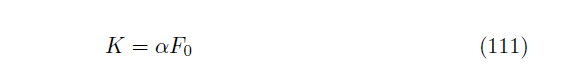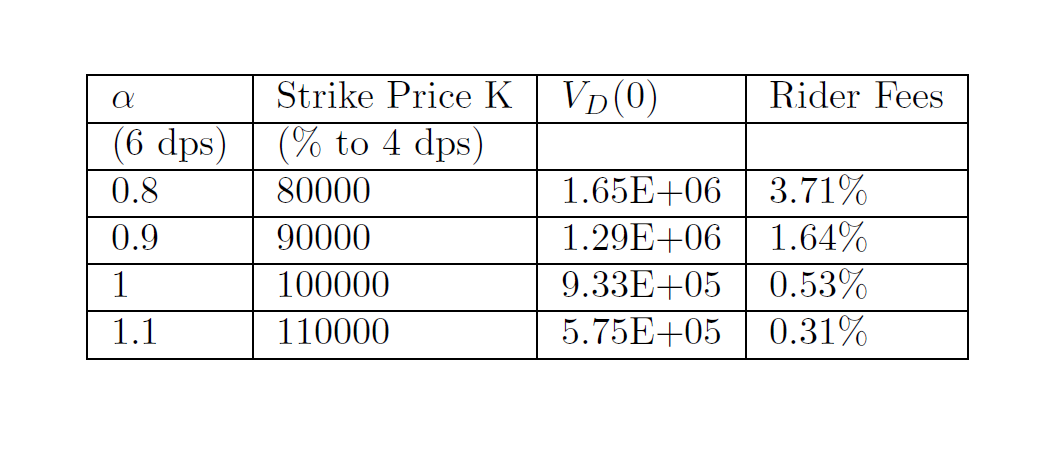• 在单元格内，需要加上括号，如下： &$$\alpha = 2.0$$ \\
在单元格内，需要加上括号，如下：
&$$\alpha = 2.0$$ \\
展开全文• 写了个表格，在插入公式的地方（第9行）总是报一堆类似的 Missing { inserted. &$C\xrightarrow{(n^',d_1^',p^')} 错误。查了好久也没解决。在今天早晨灵光乍现，突然就改好了。以下是错误代码。 \begin{... 写了个表格，在插入的公式的地方（第9行）总是报一堆类似的 Missing { inserted. &$C\xrightarrow{(n^',d_1^',p^')}

错误。查了好久也没解决。在今天早晨灵光乍现，突然就改好了。以下是错误代码。

\begin{table}[]\label{comm}
\caption{Communication cost of client and cloud/edge server in SoSQC1 and SoSQC2}
\begin{tabular}{@{}lllll@{}}

\toprule
$Algorithm$ & $Transmission\ data$ & $Data\ size$ & \multicolumn{2}{l}{$Total$}  \\
\midrule
\multirow{5}{*}{$SoSQC1$}
&$C\xrightarrow{(n^',d_1^',p^')}S$& \multicolumn{1}{l}{$6\ (Len)$} & \multirow{2}{*}{$C\to S:$} & \multirow{2}{*}{$9+2Num\ (Len)$}  \\

\multicolumn{1}{c}{}& $C\xrightarrow{(a^{'})}S$ & $2Num\ (Len)$ & \multirow{3}*{$S\to C:$} & \multirow{3}*{$2+2Num\ (Len)$}  \\
\multicolumn{1}{c}{}& $S \xrightarrow{(R_1^{'})}C$ & $2Num\ (Len)$ & & \\
\multicolumn{1}{c}{}& $C \xrightarrow{(\omega,d_2^{'})}S$ &$3\ (Len)$& & \\
\multicolumn{1}{c}{}& $S \xrightarrow{(R_2^{'})}C$ &$2\ (Len)$&  &\\
\midrule
\multirow{4}{*}{$SoSQC2$}
& $C \xrightarrow{(n^{'},d^{'},d_2^{'},p^{'})}S$ & $7\ (Len)$ & \multirow{1}{*}{$C\to S:$} &\multirow{1}{*}{$7\ (Len)$} \\
& $S \xrightarrow{(R_1^{'})}C$ & $2Num\ (Len)$ & \multirow{2}{*}{$S\to C:$} & \multirow{2}{*}{$3+2Num\ (Len)$} \\

& $S \xrightarrow{(a,R_2^{'})}C$ & $3\ (Len)$ &  &\\
\bottomrule

\end{tabular}
\end{table}

原因是你在表格中插入带有上标之类的符号不能简写。例如，平时$n^'$是没问题的，但是在表格中就会报错。修改方法就是加{}。改成： $n^{'}$ .

所以我在思考，遇到Missing { inserted.  的问题，应该好好考虑哪里缺了或者需要加 {

展开全文• 今天帮别人写Latex格式的报告，发现之前踩过的坑又踩了一遍，特此总结一下。 插公式 强烈安利Mathpix Snipping Tool，这是一款基于OCR文字识别的自动生成公式软件，节约了很多盲目打公式的时间。结合Typora也能生成...
今天帮别人写Latex格式的报告，发现之前踩过的坑又踩了一遍，特此总结一下。
插公式
强烈安利Mathpix Snipping Tool，这是一款基于OCR文字识别的自动生成公式软件，节约了很多盲目打公式的时间。结合Typora也能生成Mathtype/ Word公式等其他格式。
打公式怎么自定义编号？
	\begin{equation}
K=\alpha F_{0} \tag{111}
\end{equation}

加\tag{}即可，下面是效果插图片
怎么一行插入多张图片？
图片和文字的顺序不对？
% \usepackage{graphicx}
% \usepackage{subfigure}
\begin{figure}[H]
\centering
\subfigure{
%\label{fig:subfig:a} %% label for first subfigure
\includegraphics[width=2.3in]{Figures/11.jpg}}
\subfigure{
%\label{fig:subfig:b} %% label for second subfigure
\includegraphics[width=2.3in]{Figures/12.jpg}}
\end{figure}

这是一行两张图的效果，加\会自动换行. width如果太大也会自动换行.
\begin{figure}[H] 中的 [H] 表示它不会强制给你改变文字和图片的顺序.
插表格
插入表格也有神器，表格生成器TablesGenerator. 只需要把Excel中的表格选中，复制粘贴到生成器的表格页面，点Generate就可以生成表格对应的Latex代码了。
但是生成的表格会丢失一些竖线，有时候又需要100%还原.
表格中的横线和竖线在哪里加？
	\begin{center}
\begin{tabular}{|l|l|l|l|}
\hline
\textit{$\alpha$} & Strike Price K & \textit{$V_{D}(0)$} & Rider Fees \\ \hline
(6 dps)    & (\% to 4 dps)  &                 &            \\ \hline
0.8        & 80000          & 1.65E+06        & 3.71\%     \\ \hline
0.9        & 90000          & 1.29E+06        & 1.64\%     \\ \hline
1          & 100000         & 9.33E+05        & 0.53\%     \\ \hline
1.1        & 110000         & 5.75E+05        & 0.31\%     \\ \hline
\end{tabular}
\end{center}

效果：\begin{tabular}{|l|l|l|l|}中，字母l代表数据都是左对齐的，字母之间的分割线|就是列的竖线，如果去掉就没有竖线了.
每一行的最后写一个\ \hline，代表换行和加横线.
小细节

&，% 等符号，使用/&, /%显示
换行\\，换页\newpage
有时候公式、表格什么的连在一起，中间没有文字，觉得公式表格或者图片之间离得太近了。这是不能直接用\\，它会报错说没有文字. 这时使用~\\即可。


展开全文• 文章分两栏时图片表格以及公式如何跨栏 1，矩阵方程插入： \begin{equation} \left[ \begin{array}{cccc} {S_b}({t_1})\ {S_b}({t_2})\ … \ {S_b}({t_N}) \end{array} \right ] \left[ \begin{array}{cccc} {S_b}({...
LaTex排版技巧：
矩阵方程怎么插入
文章分两栏时图片表格以及公式如何跨栏
1，矩阵方程插入：
\begin{equation}
\left[
\begin{array}{cccc}
{S_b}({t_1})\
{S_b}({t_2})\
… \
{S_b}({t_N})
\end{array}
\right ]
\left[
\begin{array}{cccc}
{S_b}({t_1},1)& {S_b}({t_1},2) &…  & {S_b}({t_1},M)\
{S_b}({t_2},1)& {S_b}({t_2},2) &…  & {S_b}({t_2},M)\
…& … & …  &…\
{S_b}({t_N},1)& {S_b}({t_N},2) &…  &{S_b}({t_N},M)
\end{array}
\right ] \cdot
\left[
\begin{array}{cccc}
{\beta _1}\
{\beta _2}\
… \
{\beta _M}
\end{array}\right] + \left[ \begin{array}{l}
{\omega _1}\
{\omega _2}\
…\
{\omega _M}
\end{array}
\right ]
\end{equation}
2，公式跨栏插入
在头插入\begin{figure*}和尾插入\end{figure*}
3，图片和表格跨栏插入
\begin{figure*}%%加上表示跨栏插入
\vspace{-0cm}
\centering
\includegraphics[height=5.0cm,width=8cm]{Fig.12/11}
\caption{Schematic of TCAI system.}
\vspace{-0cm}
\end{figure}


展开全文• LaTeX中最实用的双栏转单栏公式表格插入插入公式插入表格 插入公式 \begin{figure*}[b] {\noindent} \rule[-10pt]{18.07cm}{0.1em} \begin{equation} e^{i\pi}+1=0 \end{equation} \end{figure*} ...
• 文章目录公式编辑器表格编辑器Latex设置表格字体大小LateX表格加标题和标签LateX插入三线表 如果在LateX中直接输入、编辑公式表格，不是不可以，但是不能直接可视化编辑，所以会比较痛苦。 以下是我常用、收藏...TeXstudio
• 学习Latex，有时不需要纠结每一行代码的含义，最好的方式就是百度到实现具体功能的代码，然后先放在ctex编译器里，再慢慢调整。...2）插入图表、公式不需要自己费心思排版，也不需要手动对图表和公式编号...排版
• 表格插入 \begin{table}[htbp] %htbp代表表格浮动位置 %表格居中 \centering %添加表头 \caption{西游记四人身份表} %创建table环境 \begin{tabular}{cccc} %4个c代表4列都居中，也可以设置l,r %表格的输入 \hline %...
• 利用Latex，即使我们不会排版和程序设计也可以生成复杂的论文规格的表格和数学公式。 我们使用matlab对数据或模型进行计算处理之后，时常会需要输出结果呈现Latex的格式，或者能方便转换成latex格式方便...matlab 数学建模 数学建模国赛
• LaTeX插图：插图的基本命令是\begin{figure}[tbp] \centering \includegraphics[width=0.7\textwidth]{图片名称} \caption{标题名称}\label{fig1} \end{figure}1.figure* 相对于通栏，figure相对于本栏。Latex总不把......